# Lesson 174. OpenGL. Model

In this lesson:

– move a separate object

In the last two lessons, we looked at two matrices.

the first (projection) Allowed us to use three-dimensional coordinates to construct the image. She took all the work of translating 3D into a flat screen image.

the other (view) Allowed camera control. We could look at the image from any angle and from any angle.

In this lesson we look at the third matrix (model) That will allow us to move, rotate, compress, and stretch individual objects in our image.

So, we have a task – to move the object in the final image. That is, you need to move only one object and not touch the other. You can usually dynamically change the vertices of this object in the array that we pass to the shader. But this is not a good way. Especially if you need to rotate the object – you have to calculate the result yourself. And in productivity we lose, if each frame we will pull all coordinates in a shader.

It is much more convenient to use the model matrix. She will just need to indicate what conversions you need, and she will calculate everything herself.

Let’s remember how everything works for us. We get one final result from several matrices. And pass it to the riding shader. And we have an array of vertices. We chase these vertices through a shader that converts them with the resultant matrix.

In the last lesson we used two matrices: projection and view. From them received the final and passed it to the shader. As a result, all objects in our image (ie, axes and triangles) passed through the shader and were converted to 2D (by projection of the part of the summary matrix) and reflected from a certain angle and at a certain angle (by view of the part of the final matrix).

In general, I lead to the fact that we used two of these matrices for all objects. And in this lesson, we will need to apply a third matrix to one of the objects (in addition to those two) in order to affect the location / size / rotation of only that object and not touch the other.

That is, projection and view will process our object along with other objects, it will be part of the overall image, and the third matrix will further transform (eg, shift or rotate) it and only it. Other objects will not be affected.

Let’s look at the code, it will become clearer with it. Download the source code and open the lesson174_model module

looking class OpenGLRenderer. The code as a whole is already familiar with past lessons. I will only comment on the changes.

We have 4 matrices described:

mProjectionMatrix – projection matrix

mViewMatrix – view matrix

mModelMatrix – model matrix

mMatrix is ​​the final matrix

In the method prepareData we define an array of vertices, in which we describe three axes and one triangle.

In the method bindMatrix we added a model matrix to the calculation of the total matrix. Multiply the view and model of the matrix, then multiply the result with the projection matrix, get the final matrix and pass it to the shader.

All the basic code from onDrawFrame for the sake of convenience and clarity, I split into two drawAxes and drawTriangle methods.

IN drawAxes we set the matrix using the setIdentityM method. Then we compute and pass the final matrix to the shader using the bindMatrix method. Since we dropped the model matrix, then multiplying it with the view matrix will give the view matrix unchanged. That is, the dropped model matrix will not affect the final matrix, which will only contain data from the view and projection of the matrices. This is exactly what we need to draw axes.

Subsequent calls to the glDrawArrays method will drive the vertices through the shader, and the shader will use the final matrix to obtain the end result.

method drawTriangle will draw a triangle. Initially, we just reset the model matrix just in case, because before we set it up, we need it clean. Next, in the setModelMatrix method, we will specify the transformations we need in the matrix model. And in the bindMatrix method, we form the final matrix and pass it to the shader. Now, the next calls to the glDrawArrays method will drive the vertices through a shader that contains a matrix constructed with a custom matrix model. Accordingly, those transformations that we put in the model matrix will be applied to the object that will be drawn by the shader. In our case, it’s a triangle.

This is probably a key point in the lesson and should be understood. That is, for the reproduction of axes, we compute the final matrix with an empty model matrix, and for the triangle with the model matrix in which we will configure the transformation. As a result, the axes will be drawn as they should, and the triangle will be displaced / rotated / compressed / stretched, looking at what transformations we will adjust in the model matrix.

method setModelMatrix is still empty. That is, we are not adjusting the model matrix yet, and the triangle will be drawn without any transformations.

run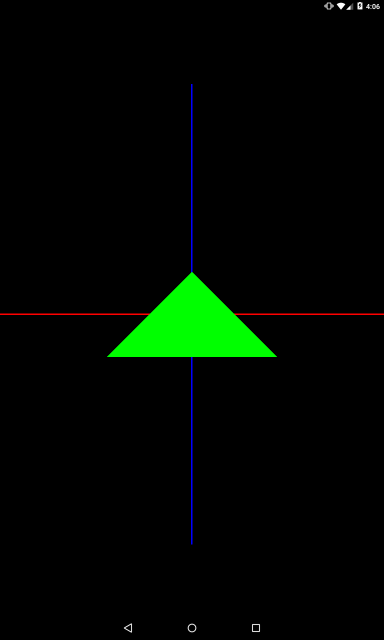### Translate

Now let’s move the triangle to the right by 1. Let’s rewrite setModelMatrix

```private void setModelMatrix() {
Matrix.translateM(mModelMatrix, 0, 1, 0, 0);
}
```

The translateM method sets the matrix to move. In it we specify the model matrix and zero indentation. The last three parameters are the offset values ​​along the X, Y, and Z axes. We set the offset along the X axis by 1. As the camera looks at images from the Z axis, then shifting the triangle along the X axis to 1 will give us a right shift.

Now, when running bindMatrix, which goes before drawing a triangle, the resulting matrix will be calculated taking into account the configured model matrix, and this will affect how the triangle is drawn – it will be shifted to the right.

run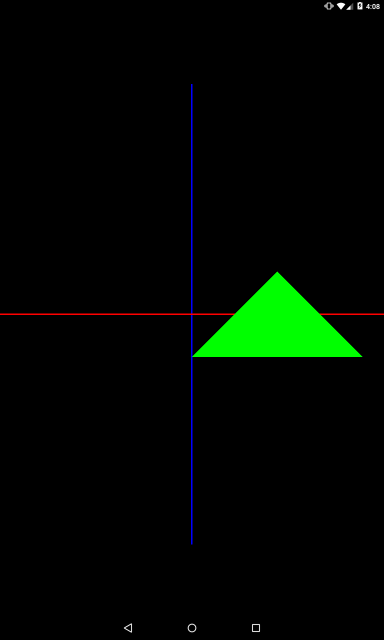The triangle is shifted to the right. Note that we did not change the initial vertices of the triangle in the array. The offset is realized by the matrix. And it was implemented only for the triangle, and the axes remained in place. It happened because different matrixes were used when displaying axes and triangles.

Let’s test further

```private void setModelMatrix() {
Matrix.translateM(mModelMatrix, 0, -1, 0, 0);
}
```

Offset by -1 on X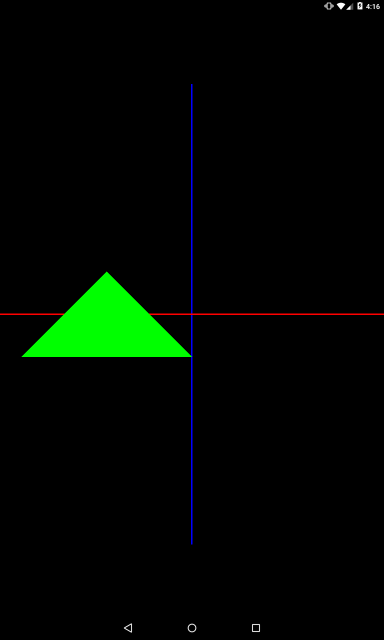```private void setModelMatrix() {
Matrix.translateM(mModelMatrix, 0, 0, 2, 0);
}
```

Y axis offset by 2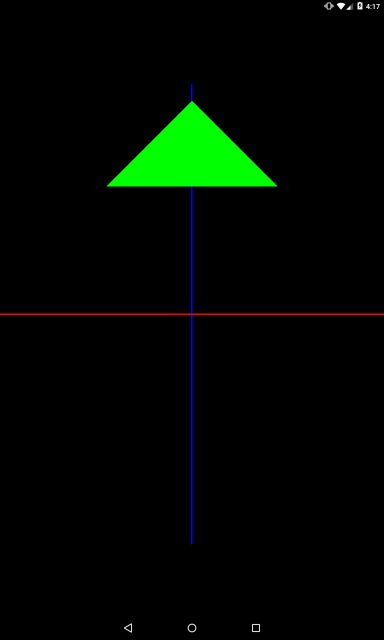```private void setModelMatrix() {
Matrix.translateM(mModelMatrix, 0, 0, 0, 2);
}
```

Z-axis offset by 2.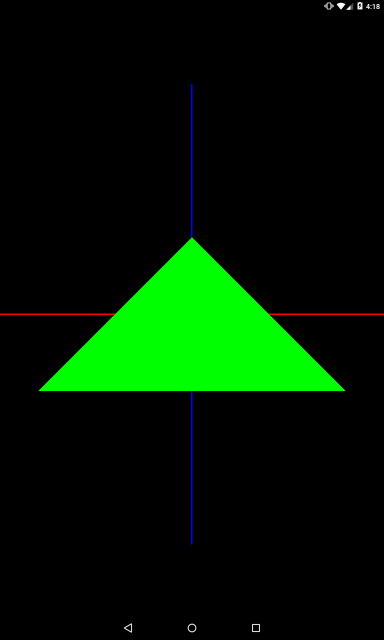Since the camera at our point (0,0,5), the triangle, shifting along the Z axis, began to be closer to the camera and began to look larger.

Now let’s give it away

```private void setModelMatrix() {
Matrix.translateM(mModelMatrix, 0, 0, 0, -1);
}
```

Z-axis offset by -1.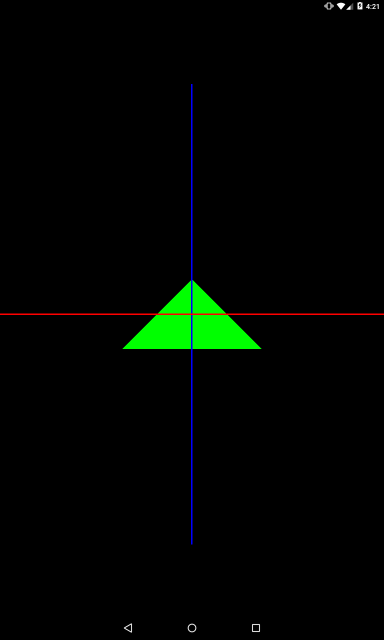The triangle became located further and it is visible that it went beyond the intersection of axes.

Of course, you can specify offset on multiple axes at once. Try it yourself.

### Scale

Consider compression / stretching capabilities. The scaleM method is similar to the translateM method, but the last three parameters are set not by the offset, but by the compression ratio for each axis.

```private void setModelMatrix() {
Matrix.scaleM(mModelMatrix, 0, 1, 1, 1);
}
```

We set three units, that is, the object will not change in any of the axes.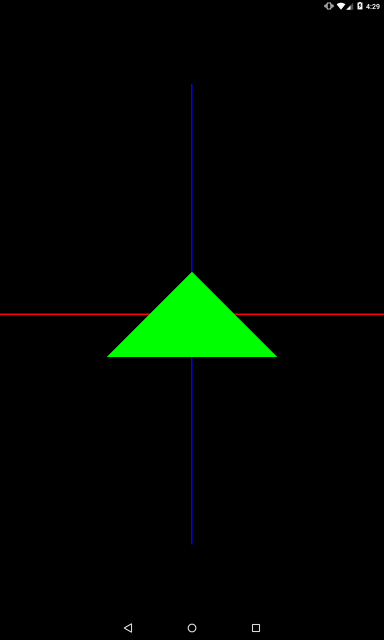```private void setModelMatrix() {
Matrix.scaleM(mModelMatrix, 0, 2, 1, 1);
}
```

We set a factor of 2 for the X axis, that is, the object will be enlarged twice along the X axis. In our example, this will be an increase in width.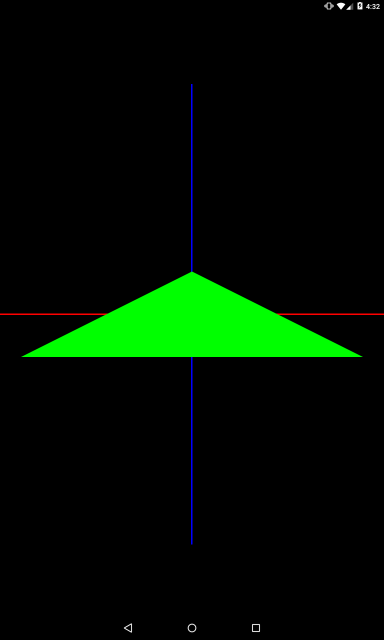```private void setModelMatrix() {
Matrix.scaleM(mModelMatrix, 0, 1, 3, 1);
}
```

Coefficient 3 on the Y axis. In our example, this will be three times the height.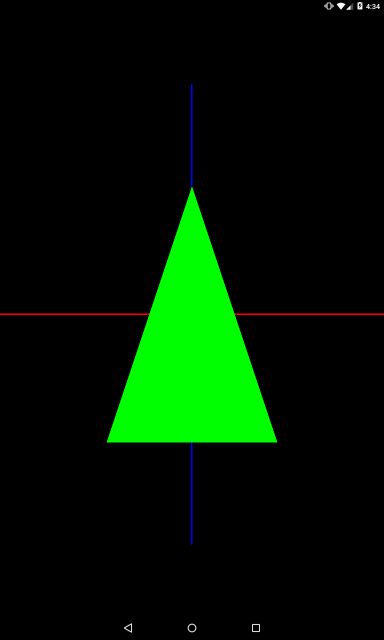If you set a factor less than one, the object will be compressed. Let’s squeeze it along the X axis.

```private void setModelMatrix() {
Matrix.scaleM(mModelMatrix, 0, 0.5f, 1, 1);
}
```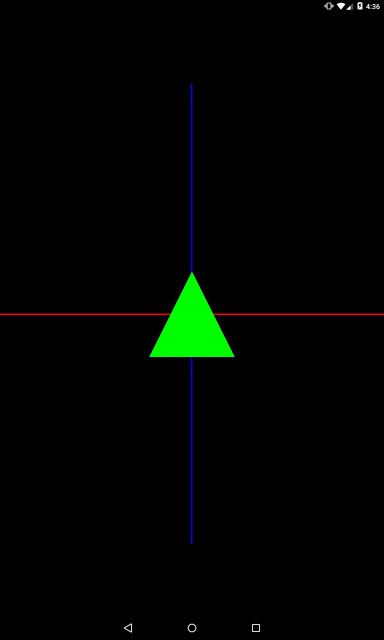If you set a negative coefficient, the object will be mirrored.

```private void setModelMatrix() {
Matrix.scaleM(mModelMatrix, 0, 1, -2, 1);
}
```

We set a factor of -2 for the Y axis. The object will be magnified twice in height and mirrored along the Y axis.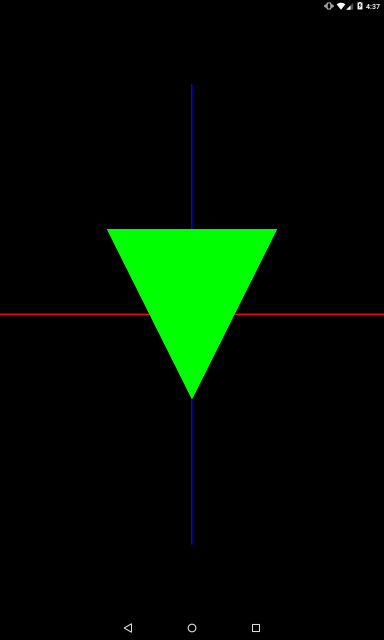You can set values ​​for multiple axes at once

```private void setModelMatrix() {
Matrix.scaleM(mModelMatrix, 0, 2, 0.5f, 1);
}
```

Stretched on the X-axis and compressed on the Y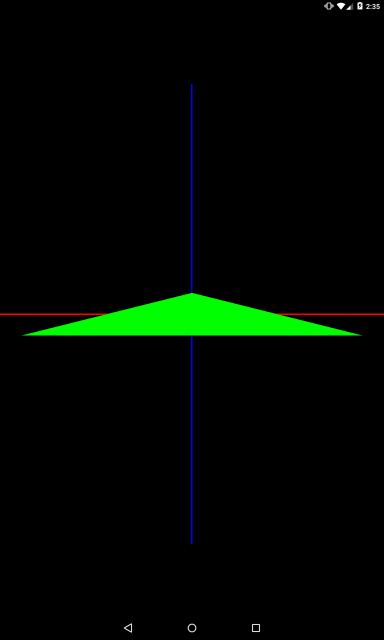### Rotate

It remains to consider the turn. To do this, we use the rotateM method, in which we specify the angle of rotation and the axis of rotation.

```private void setModelMatrix() {
Matrix.rotateM(mModelMatrix, 0, 45, 0, 0, 1);
}
```

Here we set an angle of 45 degrees and the axis of rotation – (0,0,1). That is, from the beginning of the coordinate system (point (0,0,0)), the axis is drawn through the point given by us (0,0,1). And a rotation will be made around this axis.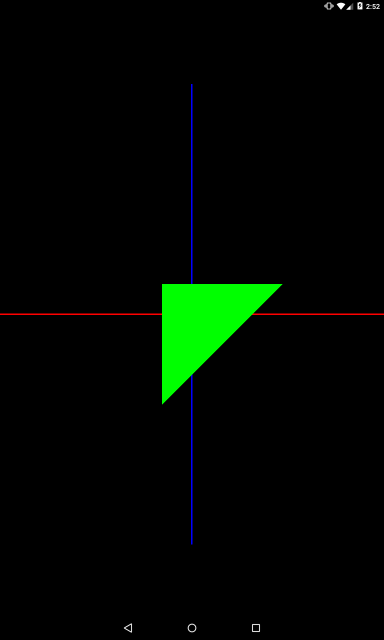The triangle turned 45 clockwise. Why against the clock?

Here is from (0,0,0) to (0,0,1) looking straight into the camera. The triangle turns 45 clockwise when viewed along the direction of this axis. As the camera looks directly opposite to the axis of rotation, it can see that the triangle has turned 45 clockwise.

Let’s change the axis direction so that it coincides with the direction of the camera. To do this, simply set the negative value to Z.

```private void setModelMatrix() {
Matrix.rotateM(mModelMatrix, 0, 45, 0, 0, -1);
}
```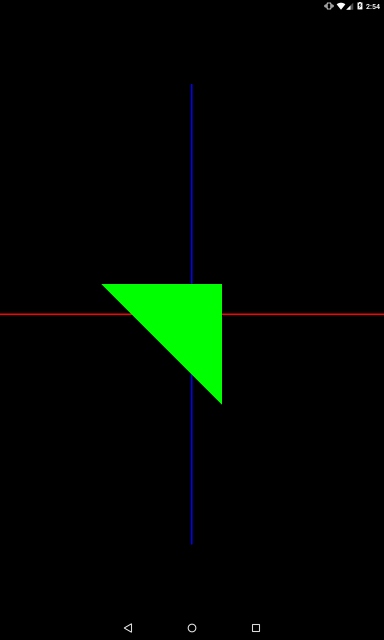Here from point (0,0,0) to point (0,0, -1) coincides with the direction of the camera and now the camera sees a clockwise turn.

Set an angle of 180 degrees

```private void setModelMatrix() {
Matrix.rotateM(mModelMatrix, 0, 180, 0, 0, -1);
}
```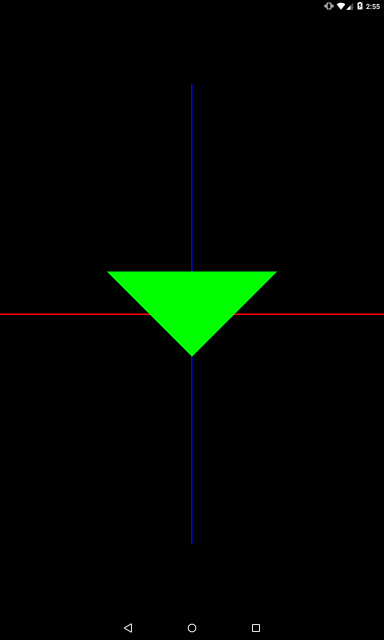The triangle turned upside down.

A little later, let’s look at the turns around the X and Y axes and add animations, but first let’s look at one important point.

You can specify more than one matrix conversion. And transformations can be both one type, and different.

For example, two resize

```private void setModelMatrix() {
Matrix.scaleM(mModelMatrix, 0, 2, 1, 1);
Matrix.scaleM(mModelMatrix, 0, 1, 0.5f, 1);
}
```

The result will be summarized.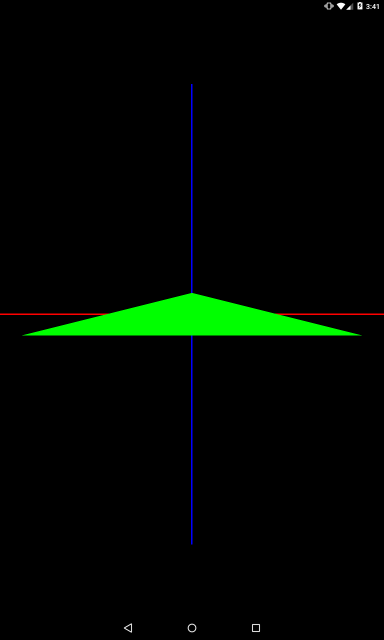The triangle also stretched twice along the X-axis and contracted to 0.5 on the Y-axis. Of course, the result will be the same if we specify both transformations in one method call at once

```private void setModelMatrix() {
Matrix.scaleM(mModelMatrix, 0, 2, 0.5f, 1);
}
```

Example with two displacements

```private void setModelMatrix() {
Matrix.translateM(mModelMatrix, 0, 1, 0, 0);
Matrix.translateM(mModelMatrix, 0, 0, 2, 0);
}
```

Both displacements will be applied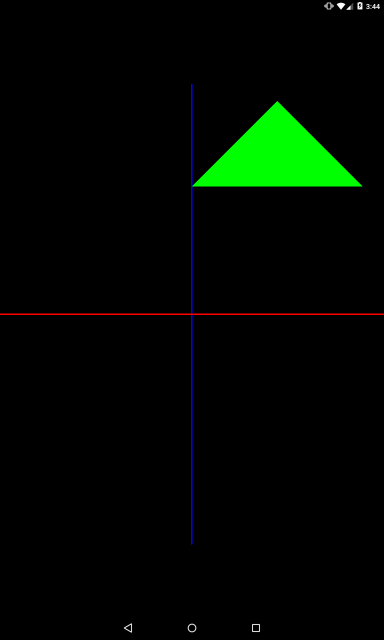The triangle will be shifted by 1 along the X axis and 2 by the Y axis.

They can also be combined into one

```private void setModelMatrix() {
Matrix.translateM(mModelMatrix, 0, 1, 2, 0);
}
```

Moving and size

```private void setModelMatrix() {
Matrix.scaleM(mModelMatrix, 0, 1, 2, 1);
Matrix.translateM(mModelMatrix, 0, 1, 0, 0);
}
```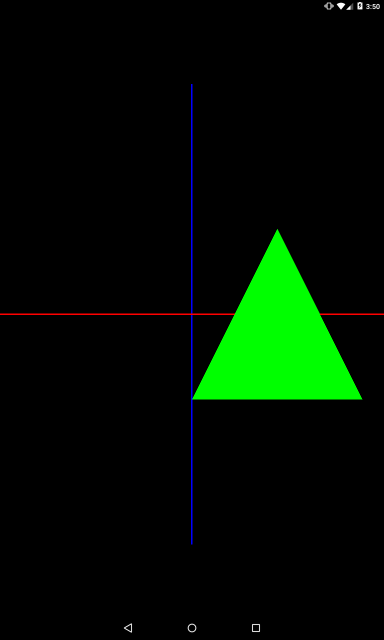The triangle is moved by 1 along the X axis and stretched twice along the Y axis

Everything is just a sample, but be careful. In some cases, the order of the transformations matters.

Consider an example: rotation + displacement

To get started, let’s move the camera a little farther from the triangle to get a bigger view. To do this, change the value in the createViewMatrix method

```float eyeZ = 8;
```

Next we set the transformation

```private void setModelMatrix() {
Matrix.translateM(mModelMatrix, 0, 2f, 0, 0);
Matrix.rotateM(mModelMatrix, 0, 45, 0, 0, 1);
}
```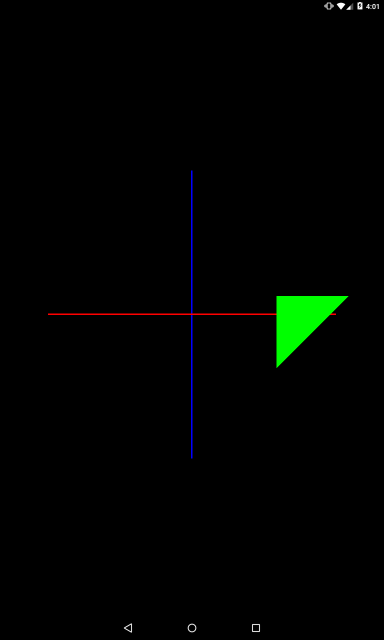We see that the triangle is rotated 45 degrees around the Z axis and displaced 2 by the X axis.

That is, the first turn was made, then the move.

Change places of conversion:

```private void setModelMatrix() {
Matrix.rotateM(mModelMatrix, 0, 45, 0, 0, 1);
Matrix.translateM(mModelMatrix, 0, 2f, 0, 0);
}
```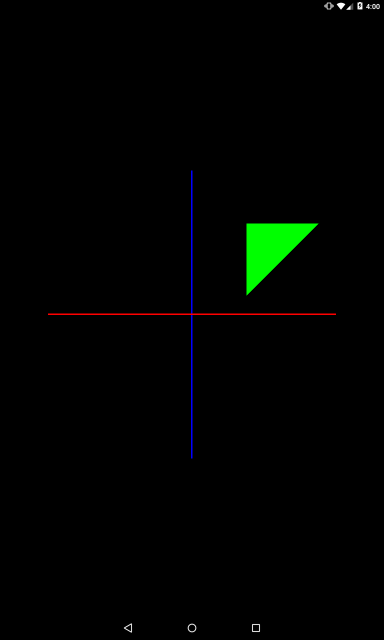It would seem that the same operations and the result is different. This is because the order of operations has changed. Initially, the triangle was shifted by 2 along the X axis. Then from that position it was rotated about the Z axis and went up accordingly.

That is, it seems that the operations are performed in reverse order because we specify in the code. Consider this moment when you are making several transformations.

```private void setModelMatrix() {
float angle = (float)(SystemClock.uptimeMillis() % TIME) / TIME * 360;
Matrix.rotateM(mModelMatrix, 0, angle, 0, 0, 1);
Matrix.translateM(mModelMatrix, 0, 2f, 0, 0);
}
```

The angle angle will change from 0 to 360 every 10 seconds.The animation clearly shows that the triangle moves each frame first along the X axis and then rotates around the Z axis.

We swap the conversion operations again to see what it will look like in the animation

```private void setModelMatrix() {
float angle = (float)(SystemClock.uptimeMillis() % TIME) / TIME * 360;
Matrix.translateM(mModelMatrix, 0, 2f, 0, 0);
Matrix.rotateM(mModelMatrix, 0, angle, 0, 0, 1);
}
```It is already evident that each frame of the triangle first rotates around the Z axis, and then we shift it along the X axis to the right.

Once again I will write about just in case what is happening at all.

1) In the prepareData method, we have prepared vertex data in the array, and in the bindData method we have passed them to vertex shaders. We were called these methods once at the beginning, in the onSurfaceCreated method. That is, we only transmit vertices once, not every frame!

2) When displaying each frame, the system calls the onDrawFrame method. In it, we call glDrawArrays, in which we specify which vertices (from the ones we passed in paragraph 1) the shader should take and which one we draw from them.

3) In addition, onDrawFrame we list the matrix and pass it to the shader. That is, we do this more than once at the very beginning, as vertices, namely each frame. And so we frame each model with a rotateM method and a constantly changing variable angle, each new frame of the summary matrix contains data that is different from the data it contained when the previous frame was played.

4) As a result, each frame shader takes the data we transmitted to it in paragraph 1, applies to them the resulting matrix in the current frame and gives the calculated coordinates of the vertices. As a result, each new frame of the object is drawn in a different place and that is what gives us the animation.

Now you can go back to the turn and look at it in more detail.

We slightly shift the camera to see the triangle at an angle. This will make it better to see the turns on all axes. To do this, change the camera position in the createViewMatrix method

```float eyeX = 2;
float eyeY = 2;
float eyeZ = 3;
```

And in the transformation we will set a turn

```private void setModelMatrix() {
float angle = (float)(SystemClock.uptimeMillis() % TIME) / TIME * 360;
Matrix.rotateM(mModelMatrix, 0, angle, 0, 0, -1);
}
```We see a rotation around the Z axis (yellow). Let’s also try the X and Y axes.

In the setModelMatrix method, change the parameters of the rotateM method:

```Matrix.rotateM(mModelMatrix, 0, angle, 1, 0, 0);
```

The triangle will now rotate around the X axis (red)Y-axis (blue)

```Matrix.rotateM(mModelMatrix, 0, angle, 0, 1, 0);
```Now the axis drawn to the point (0,1,1)

```Matrix.rotateM(mModelMatrix, 0, angle, 0, 1, 1);
```

Try to imagine an axis going from point (0,0,0) to point (0,1,1) – that is, it will lie between the axes Y and Z. And a triangle will rotate around it.As a small independent work, try to display the axis of rotation, just as we output the up-vector in the last lesson. This will give more clarity of rotation.

Discuss in the forum [9 replies]